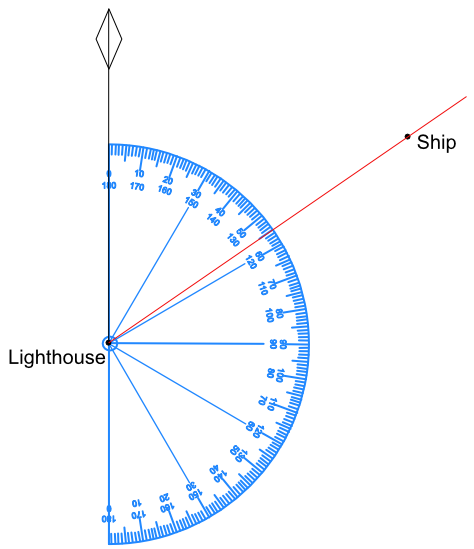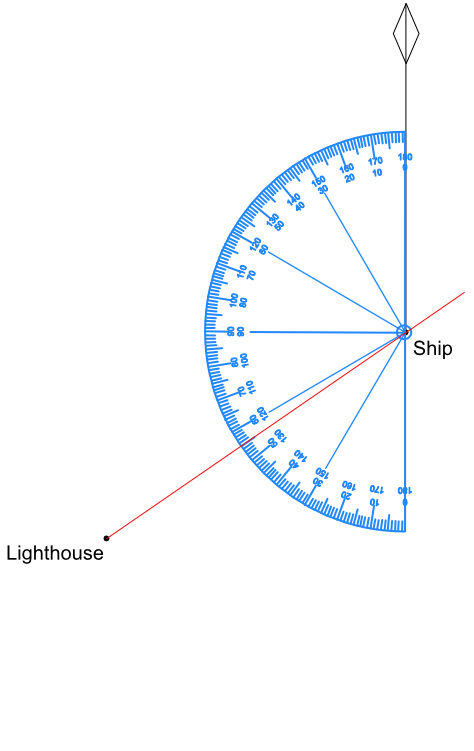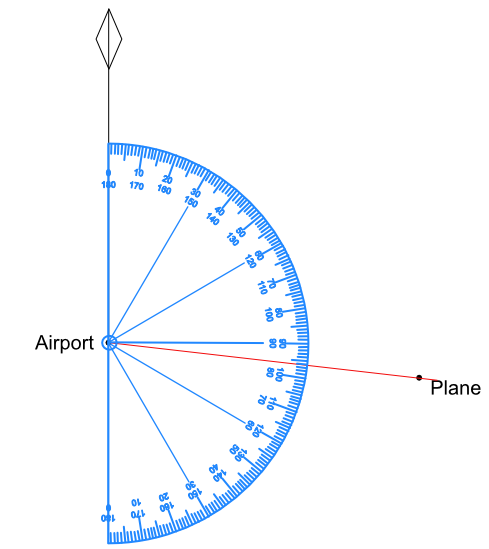Bearings

# Bearings

GCSE(F),

A Bearing is the amount of turn clockwise from the North direction. The amount of turn is given as an angle and in degrees.

North is shown with a vertical line. At the top of the line is either a diamond shape or the letter N.

A bearing is always given as a three-digit number. For numbers less than 100, use zeroes (0) at the front of the number to make three digits.

A bearing is described as to a point from a point; for example:
the bearing to a ship from a lighthouse is 055º

The angle is measured at the lighthouse (the from), and is the angle between North and the ship:A reciprocal bearing is a bearing taken from the other point. A reciprocal bearing is 180º different to the original bearing.

## Examples

1. What is the bearing of the lighthouse from the ship?This is a line from the ship to the lighthouse. Measure the angle between North and the lighthouse.

Because the angle measured is anticlockwise, subtract this angle from 360º for the clockwise angle: 360 - 125 = 235º2. What is the bearing of the plane from the airport?